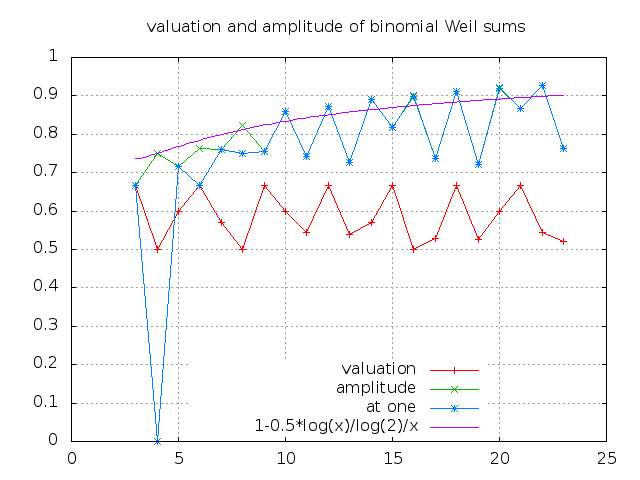# Binomial Weil Sums

This page reports the numerical experiments (Summer 2016) on Weil sums.

### Definitions and notations

Let m be a positive integer, X a nontrivial additive character of GF(2,m). We consider the Weil sum

W(s,a) := sum_{x in GF(2,m)} X( x^s + ax ).

one defines the valuation of s

val(s) := min_{a!=0} dyadic valuation of W(s,a)

and spectral amplitude of s by
spec(s) := max_{a} absolute value of W(s,a)

The goal of the project is to compute the distribution of valuations and spectral amplitudes when s ranges among the invertible exponents, for m smaller or equal to 32.

### picture

In the picture below, we plot the graphs of the quantities :

m -> log_q max_s spec(s) m -> log_q max_s abs( walsh(s, 1) ) m -> log_q max_s val(s)
where q:=2^m### Interpretation

Actually, we have fully understood the non-archimedian profile.

It seems enough to understand the Fourier coefficient at one.

### Methodology

Philippe Langevin, Summer 2016